Algebra and Trigonometry

# 5.3Graphs of Polynomial Functions

Algebra and Trigonometry5.3 Graphs of Polynomial Functions

### Learning Objectives

In this section, you will:

• Recognize characteristics of graphs of polynomial functions.
• Use factoring to ﬁnd zeros of polynomial functions.
• Identify zeros and their multiplicities.
• Determine end behavior.
• Understand the relationship between degree and turning points.
• Graph polynomial functions.
• Use the Intermediate Value Theorem.

The revenue in millions of dollars for a fictional cable company from 2006 through 2013 is shown in Table 1.

 Year 2006 2007 2008 2009 2010 2011 2012 2013 Revenues 52.4 52.8 51.2 49.5 48.6 48.6 48.7 47.1
Table 1

The revenue can be modeled by the polynomial function

$R(t)=−0.037 t 4 +1.414 t 3 −19.777 t 2 +118.696t−205.332 R(t)=−0.037 t 4 +1.414 t 3 −19.777 t 2 +118.696t−205.332$

where $R R$ represents the revenue in millions of dollars and $t t$ represents the year, with $t=6 t=6$ corresponding to 2006. Over which intervals is the revenue for the company increasing? Over which intervals is the revenue for the company decreasing? These questions, along with many others, can be answered by examining the graph of the polynomial function. We have already explored the local behavior of quadratics, a special case of polynomials. In this section we will explore the local behavior of polynomials in general.

### Recognizing Characteristics of Graphs of Polynomial Functions

Polynomial functions of degree 2 or more have graphs that do not have sharp corners; recall that these types of graphs are called smooth curves. Polynomial functions also display graphs that have no breaks. Curves with no breaks are called continuous. Figure 1 shows a graph that represents a polynomial function and a graph that represents a function that is not a polynomial.

Figure 1

### Example 1

#### Recognizing Polynomial Functions

Which of the graphs in Figure 2 represents a polynomial function?

Figure 2

### Q&A

Do all polynomial functions have as their domain all real numbers?

Yes. Any real number is a valid input for a polynomial function.

### Using Factoring to Find Zeros of Polynomial Functions

Recall that if $f f$ is a polynomial function, the values of $x x$ for which $f( x )=0 f( x )=0$ are called zeros of $f. f.$ If the equation of the polynomial function can be factored, we can set each factor equal to zero and solve for the zeros.

We can use this method to find $x- x-$ intercepts because at the $x- x-$ intercepts we find the input values when the output value is zero. For general polynomials, this can be a challenging prospect. While quadratics can be solved using the relatively simple quadratic formula, the corresponding formulas for cubic and fourth-degree polynomials are not simple enough to remember, and formulas do not exist for general higher-degree polynomials. Consequently, we will limit ourselves to three cases:

1. The polynomial can be factored using known methods: greatest common factor and trinomial factoring.
2. The polynomial is given in factored form.
3. Technology is used to determine the intercepts.

### How To

Given a polynomial function $f, f,$ find the x-intercepts by factoring.

1. Set $f( x )=0. f( x )=0.$
2. If the polynomial function is not given in factored form:
1. Factor out any common monomial factors.
2. Factor any factorable binomials or trinomials.
3. Set each factor equal to zero and solve to find the $x- x-$ intercepts.

### Example 2

#### Finding the x-Intercepts of a Polynomial Function by Factoring

Find the x-intercepts of $f(x)= x 6 −3 x 4 +2 x 2 . f(x)= x 6 −3 x 4 +2 x 2 .$

### Example 3

#### Finding the x-Intercepts of a Polynomial Function by Factoring

Find the x-intercepts of $f(x)= x 3 −5 x 2 −x+5. f(x)= x 3 −5 x 2 −x+5.$

### Example 4

#### Finding the y- and x-Intercepts of a Polynomial in Factored Form

Find the y- and x-intercepts of $g(x)= (x−2) 2 (2x+3). g(x)= (x−2) 2 (2x+3).$

#### Analysis

We can always check that our answers are reasonable by using a graphing calculator to graph the polynomial as shown in Figure 5.

Figure 5

### Example 5

#### Finding the x-Intercepts of a Polynomial Function Using a Graph

Find the x-intercepts of $h(x)= x 3 +4 x 2 +x−6. h(x)= x 3 +4 x 2 +x−6.$

### Try It #1

Find the y- and x-intercepts of the function $f(x)= x 4 −19 x 2 +30x. f(x)= x 4 −19 x 2 +30x.$

### Identifying Zeros and Their Multiplicities

Graphs behave differently at various x-intercepts. Sometimes, the graph will cross over the horizontal axis at an intercept. Other times, the graph will touch the horizontal axis and "bounce" off.

Suppose, for example, we graph the function shown.

$f(x)=(x+3) (x−2) 2 (x+1) 3 f(x)=(x+3) (x−2) 2 (x+1) 3$

Notice in Figure 7 that the behavior of the function at each of the x-intercepts is different.

Figure 7 Identifying the behavior of the graph at an x-intercept by examining the multiplicity of the zero.

The x-intercept $x=−3 x=−3$ is the solution of equation $(x+3)=0. (x+3)=0.$ The graph passes directly through the x-intercept at $x=−3. x=−3.$ The factor is linear (has a degree of 1), so the behavior near the intercept is like that of a line—it passes directly through the intercept. We call this a single zero because the zero corresponds to a single factor of the function.

The x-intercept $x=2 x=2$ is the repeated solution of equation $(x−2) 2 =0. (x−2) 2 =0.$ The graph touches the axis at the intercept and changes direction. The factor is quadratic (degree 2), so the behavior near the intercept is like that of a quadratic—it bounces off of the horizontal axis at the intercept.

$(x−2) 2 =(x−2)(x−2) (x−2) 2 =(x−2)(x−2)$

The factor is repeated, that is, the factor $( x−2 ) ( x−2 )$ appears twice. The number of times a given factor appears in the factored form of the equation of a polynomial is called the multiplicity. The zero associated with this factor, $x=2, x=2,$ has multiplicity 2 because the factor $( x−2 ) ( x−2 )$ occurs twice.

The x-intercept $x=−1 x=−1$ is the repeated solution of factor $(x+1) 3 =0. (x+1) 3 =0.$ The graph passes through the axis at the intercept, but flattens out a bit first. This factor is cubic (degree 3), so the behavior near the intercept is like that of a cubic—with the same S-shape near the intercept as the toolkit function $f( x )= x 3 . f( x )= x 3 .$ We call this a triple zero, or a zero with multiplicity 3.

For zeros with even multiplicities, the graphs touch or are tangent to the x-axis. For zeros with odd multiplicities, the graphs cross or intersect the x-axis. See Figure 8 for examples of graphs of polynomial functions with multiplicity 1, 2, and 3.

Figure 8

For higher even powers, such as 4, 6, and 8, the graph will still touch and bounce off of the horizontal axis but, for each increasing even power, the graph will appear flatter as it approaches and leaves the x-axis.

For higher odd powers, such as 5, 7, and 9, the graph will still cross through the horizontal axis, but for each increasing odd power, the graph will appear flatter as it approaches and leaves the x-axis.

### Graphical Behavior of Polynomials at x-Intercepts

If a polynomial contains a factor of the form $(x−h) p , (x−h) p ,$ the behavior near the $x- x-$ intercept $h h$ is determined by the power $p. p.$ We say that $x=h x=h$ is a zero of multiplicity $p. p.$

The graph of a polynomial function will touch the x-axis at zeros with even multiplicities. The graph will cross the x-axis at zeros with odd multiplicities.

The sum of the multiplicities is the degree of the polynomial function.

### How To

Given a graph of a polynomial function of degree $n, n,$ identify the zeros and their multiplicities.

1. If the graph crosses the x-axis and appears almost linear at the intercept, it is a single zero.
2. If the graph touches the x-axis and bounces off of the axis, it is a zero with even multiplicity.
3. If the graph crosses the x-axis at a zero, it is a zero with odd multiplicity.
4. The sum of the multiplicities is $n. n.$

### Example 6

#### Identifying Zeros and Their Multiplicities

Use the graph of the function of degree 6 in Figure 9 to identify the zeros of the function and their possible multiplicities.

Figure 9

### Try It #2

Use the graph of the function of degree 9 in Figure 10 to identify the zeros of the function and their multiplicities.

Figure 10

### Determining End Behavior

As we have already learned, the behavior of a graph of a polynomial function of the form

$f(x)= a n x n + a n−1 x n−1 +...+ a 1 x+ a 0 f(x)= a n x n + a n−1 x n−1 +...+ a 1 x+ a 0$

will either ultimately rise or fall as $x x$ increases without bound and will either rise or fall as $x x$ decreases without bound. This is because for very large inputs, say 100 or 1,000, the leading term dominates the size of the output. The same is true for very small inputs, say –100 or –1,000.

Recall that we call this behavior the end behavior of a function. As we pointed out when discussing quadratic equations, when the leading term of a polynomial function, $a n x n , a n x n ,$ is an even power function, as $x x$ increases or decreases without bound, $f(x) f(x)$ increases without bound. When the leading term is an odd power function, as $x x$ decreases without bound, $f(x) f(x)$ also decreases without bound; as $x x$ increases without bound, $f(x) f(x)$ also increases without bound. If the leading term is negative, it will change the direction of the end behavior. Figure 11 summarizes all four cases.

Figure 11

### Understanding the Relationship between Degree and Turning Points

In addition to the end behavior, recall that we can analyze a polynomial function’s local behavior. It may have a turning point where the graph changes from increasing to decreasing (rising to falling) or decreasing to increasing (falling to rising). Look at the graph of the polynomial function $f(x)= x 4 − x 3 −4 x 2 +4x f(x)= x 4 − x 3 −4 x 2 +4x$ in Figure 12. The graph has three turning points.

Figure 12

This function $f f$ is a 4th degree polynomial function and has 3 turning points. The maximum number of turning points of a polynomial function is always one less than the degree of the function.

### Interpreting Turning Points

A turning point is a point of the graph where the graph changes from increasing to decreasing (rising to falling) or decreasing to increasing (falling to rising).

A polynomial of degree $n n$ will have at most $n−1 n−1$ turning points.

### Example 7

#### Finding the Maximum Number of Turning Points Using the Degree of a Polynomial Function

Find the maximum number of turning points of each polynomial function.

1. $f(x)=− x 3 +4 x 5 −3 x 2 +1 f(x)=− x 3 +4 x 5 −3 x 2 +1$
2. $f(x)=− ( x−1 ) 2 ( 1+2 x 2 ) f(x)=− ( x−1 ) 2 ( 1+2 x 2 )$

### Graphing Polynomial Functions

We can use what we have learned about multiplicities, end behavior, and turning points to sketch graphs of polynomial functions. Let us put this all together and look at the steps required to graph polynomial functions.

### How To

Given a polynomial function, sketch the graph.

1. Find the intercepts.
2. Check for symmetry. If the function is an even function, its graph is symmetrical about the $y- y-$ axis, that is, $f( −x )=f( x ). f( −x )=f( x ).$ If a function is an odd function, its graph is symmetrical about the origin, that is, $f( −x )=−f( x ). f( −x )=−f( x ).$
3. Use the multiplicities of the zeros to determine the behavior of the polynomial at the $x- x-$ intercepts.
4. Determine the end behavior by examining the leading term.
5. Use the end behavior and the behavior at the intercepts to sketch a graph.
6. Ensure that the number of turning points does not exceed one less than the degree of the polynomial.
7. Optionally, use technology to check the graph.

### Example 8

#### Sketching the Graph of a Polynomial Function

Sketch a graph of $f(x)=−2 (x+3) 2 (x−5). f(x)=−2 (x+3) 2 (x−5).$

### Try It #3

Sketch a graph of $f(x)= 1 4 x (x−1) 4 (x+3) 3 . f(x)= 1 4 x (x−1) 4 (x+3) 3 .$

### Using the Intermediate Value Theorem

In some situations, we may know two points on a graph but not the zeros. If those two points are on opposite sides of the x-axis, we can confirm that there is a zero between them. Consider a polynomial function $f f$ whose graph is smooth and continuous. The Intermediate Value Theorem states that for two numbers $a a$ and $b b$ in the domain of $f, f,$ if $a and $f( a )≠f( b ), f( a )≠f( b ),$ then the function $f f$ takes on every value between $f( a ) f( a )$ and $f( b ). f( b ).$ (While the theorem is intuitive, the proof is actually quite complicated and requires higher mathematics.) We can apply this theorem to a special case that is useful in graphing polynomial functions. If a point on the graph of a continuous function $f f$ at $x=a x=a$ lies above the $x- x-$ axis and another point at $x=b x=b$ lies below the $x- x-$ axis, there must exist a third point between $x=a x=a$ and $x=b x=b$ where the graph crosses the $x- x-$ axis. Call this point $( c,f( c ) ). ( c,f( c ) ).$ This means that we are assured there is a solution $c c$ where $f( c )=0. f( c )=0.$

In other words, the Intermediate Value Theorem tells us that when a polynomial function changes from a negative value to a positive value, the function must cross the $x- x-$ axis. Figure 17 shows that there is a zero between $a a$ and $b. b.$

Figure 17 Using the Intermediate Value Theorem to show there exists a zero.

### Intermediate Value Theorem

Let $f f$ be a polynomial function. The Intermediate Value Theorem states that if $f( a ) f( a )$ and $f( b ) f( b )$ have opposite signs, then there exists at least one value $c c$ between $a a$ and $b b$ for which $f( c )=0. f( c )=0.$

### Example 9

#### Using the Intermediate Value Theorem

Show that the function $f(x)= x 3 −5 x 2 +3x+6 f(x)= x 3 −5 x 2 +3x+6$ has at least two real zeros between $x=1 x=1$ and $x=4. x=4.$

#### Analysis

We can also see on the graph of the function in Figure 18 that there are two real zeros between $x=1 x=1$ and $x=4. x=4.$

Figure 18

### Try It #4

Show that the function $f(x)=7 x 5 −9 x 4 − x 2 f(x)=7 x 5 −9 x 4 − x 2$ has at least one real zero between $x=1 x=1$ and $x=2. x=2.$

#### Writing Formulas for Polynomial Functions

Now that we know how to find zeros of polynomial functions, we can use them to write formulas based on graphs. Because a polynomial function written in factored form will have an x-intercept where each factor is equal to zero, we can form a function that will pass through a set of x-intercepts by introducing a corresponding set of factors.

### Factored Form of Polynomials

If a polynomial of lowest degree $p p$ has horizontal intercepts at $x= x 1 , x 2 ,…, x n , x= x 1 , x 2 ,…, x n ,$ then the polynomial can be written in the factored form: $f(x)=a (x− x 1 ) p 1 (x− x 2 ) p 2 ⋯ (x− x n ) p n f(x)=a (x− x 1 ) p 1 (x− x 2 ) p 2 ⋯ (x− x n ) p n$ where the powers $p i p i$ on each factor can be determined by the behavior of the graph at the corresponding intercept, and the stretch factor $a a$ can be determined given a value of the function other than the x-intercept.

### How To

Given a graph of a polynomial function, write a formula for the function.

1. Identify the x-intercepts of the graph to find the factors of the polynomial.
2. Examine the behavior of the graph at the x-intercepts to determine the multiplicity of each factor.
3. Find the polynomial of least degree containing all the factors found in the previous step.
4. Use any other point on the graph (the y-intercept may be easiest) to determine the stretch factor.

### Example 10

#### Writing a Formula for a Polynomial Function from the Graph

Write a formula for the polynomial function shown in Figure 19.

Figure 19

### Try It #5

Given the graph shown in Figure 20, write a formula for the function shown.

Figure 20

#### Using Local and Global Extrema

With quadratics, we were able to algebraically find the maximum or minimum value of the function by finding the vertex. For general polynomials, finding these turning points is not possible without more advanced techniques from calculus. Even then, finding where extrema occur can still be algebraically challenging. For now, we will estimate the locations of turning points using technology to generate a graph.

Each turning point represents a local minimum or maximum. Sometimes, a turning point is the highest or lowest point on the entire graph. In these cases, we say that the turning point is a global maximum or a global minimum. These are also referred to as the absolute maximum and absolute minimum values of the function.

### Local and Global Extrema

A local maximum or local minimum at $x=a x=a$ (sometimes called the relative maximum or minimum, respectively) is the output at the highest or lowest point on the graph in an open interval around $x=a. x=a.$ If a function has a local maximum at $a, a,$ then $f(a)≥f(x) f(a)≥f(x)$ for all $x x$ in an open interval around $x=a. x=a.$ If a function has a local minimum at $a, a,$ then $f(a)≤f(x) f(a)≤f(x)$ for all $x x$ in an open interval around $x=a. x=a.$

A global maximum or global minimum is the output at the highest or lowest point of the function. If a function has a global maximum at $a, a,$ then $f(a)≥f(x) f(a)≥f(x)$ for all $x. x.$ If a function has a global minimum at $a, a,$ then $f(a)≤f(x) f(a)≤f(x)$ for all $x. x.$

We can see the difference between local and global extrema in Figure 21.

Figure 21

### Q&A

Do all polynomial functions have a global minimum or maximum?

No. Only polynomial functions of even degree have a global minimum or maximum. For example, $f( x )=x f( x )=x$ has neither a global maximum nor a global minimum.

### Example 11

#### Using Local Extrema to Solve Applications

An open-top box is to be constructed by cutting out squares from each corner of a 14 cm by 20 cm sheet of plastic and then folding up the sides. Find the size of squares that should be cut out to maximize the volume enclosed by the box.

### Try It #6

Use technology to find the maximum and minimum values on the interval $[−1,4] [−1,4]$ of the function $f(x)=−0.2 (x−2) 3 (x+1) 2 (x−4). f(x)=−0.2 (x−2) 3 (x+1) 2 (x−4).$

### Media

Access the following online resource for additional instruction and practice with graphing polynomial functions.

### 5.3 Section Exercises

#### Verbal

1.

What is the difference between an $x- x-$ intercept and a zero of a polynomial function $f? f?$

2.

If a polynomial function of degree $n n$ has $n n$ distinct zeros, what do you know about the graph of the function?

3.

Explain how the Intermediate Value Theorem can assist us in finding a zero of a function.

4.

Explain how the factored form of the polynomial helps us in graphing it.

5.

If the graph of a polynomial just touches the x-axis and then changes direction, what can we conclude about the factored form of the polynomial?

#### Algebraic

For the following exercises, find the $x- x-$ or t-intercepts of the polynomial functions.

6.

$C( t )=2( t−4 )( t+1 )(t−6) C( t )=2( t−4 )( t+1 )(t−6)$

7.

$C( t )=3( t+2 )( t−3 )(t+5) C( t )=3( t+2 )( t−3 )(t+5)$

8.

$C( t )=4t ( t−2 ) 2 (t+1) C( t )=4t ( t−2 ) 2 (t+1)$

9.

$C( t )=2t( t−3 ) ( t+1 ) 2 C( t )=2t( t−3 ) ( t+1 ) 2$

10.

$C( t )=2 t 4 −8 t 3 +6 t 2 C( t )=2 t 4 −8 t 3 +6 t 2$

11.

$C( t )=4 t 4 +12 t 3 −40 t 2 C( t )=4 t 4 +12 t 3 −40 t 2$

12.

$f(x)= x 4 − x 2 f(x)= x 4 − x 2$

13.

$f(x)= x 3 + x 2 −20x f(x)= x 3 + x 2 −20x$

14.

$f(x)= x 3 +6 x 2 −7x f(x)= x 3 +6 x 2 −7x$

15.

$f(x)= x 3 + x 2 −4x−4 f(x)= x 3 + x 2 −4x−4$

16.

$f(x)= x 3 +2 x 2 −9x−18 f(x)= x 3 +2 x 2 −9x−18$

17.

$f(x)=2 x 3 − x 2 −8x+4 f(x)=2 x 3 − x 2 −8x+4$

18.

$f(x)= x 6 −7 x 3 −8 f(x)= x 6 −7 x 3 −8$

19.

$f(x)=2 x 4 +6 x 2 −8 f(x)=2 x 4 +6 x 2 −8$

20.

$f(x)= x 3 −3 x 2 −x+3 f(x)= x 3 −3 x 2 −x+3$

21.

$f(x)= x 6 −2 x 4 −3 x 2 f(x)= x 6 −2 x 4 −3 x 2$

22.

$f(x)= x 6 −3 x 4 −4 x 2 f(x)= x 6 −3 x 4 −4 x 2$

23.

$f(x)= x 5 −5 x 3 +4x f(x)= x 5 −5 x 3 +4x$

For the following exercises, use the Intermediate Value Theorem to confirm that the given polynomial has at least one zero within the given interval.

24.

$f(x)= x 3 −9x, f(x)= x 3 −9x,$ between $x=−4 x=−4$ and $x=−2. x=−2.$

25.

$f(x)= x 3 −9x, f(x)= x 3 −9x,$ between $x=2 x=2$ and $x=4. x=4.$

26.

$f(x)= x 5 −2x, f(x)= x 5 −2x,$ between $x=1 x=1$ and $x=2. x=2.$

27.

$f(x)=− x 4 +4, f(x)=− x 4 +4,$ between $x=1 x=1$ and $x=3 x=3$ .

28.

$f(x)=−2 x 3 −x, f(x)=−2 x 3 −x,$ between $x=–1 x=–1$ and $x=1. x=1.$

29.

$f(x)= x 3 −100x+2, f(x)= x 3 −100x+2,$ between $x=0.01 x=0.01$ and $x=0.1 x=0.1$

For the following exercises, find the zeros and give the multiplicity of each.

30.

$f(x)= ( x+2 ) 3 ( x−3 ) 2 f(x)= ( x+2 ) 3 ( x−3 ) 2$

31.

$f(x)= x 2 ( 2x+3 ) 5 ( x−4 ) 2 f(x)= x 2 ( 2x+3 ) 5 ( x−4 ) 2$

32.

$f(x)= x 3 ( x−1 ) 3 ( x+2 ) f(x)= x 3 ( x−1 ) 3 ( x+2 )$

33.

$f(x)= x 2 ( x 2 +4x+4 ) f(x)= x 2 ( x 2 +4x+4 )$

34.

$f(x)= ( 2x+1 ) 3 ( 9 x 2 −6x+1 ) f(x)= ( 2x+1 ) 3 ( 9 x 2 −6x+1 )$

35.

$f(x)= ( 3x+2 ) 5 ( x 2 −10x+25 ) f(x)= ( 3x+2 ) 5 ( x 2 −10x+25 )$

36.

$f(x)=x( 4 x 2 −12x+9 )( x 2 +8x+16 ) f(x)=x( 4 x 2 −12x+9 )( x 2 +8x+16 )$

37.

$f(x)= x 6 − x 5 −2 x 4 f(x)= x 6 − x 5 −2 x 4$

38.

$f(x)=3 x 4 +6 x 3 +3 x 2 f(x)=3 x 4 +6 x 3 +3 x 2$

39.

$f(x)=4 x 5 −12 x 4 +9 x 3 f(x)=4 x 5 −12 x 4 +9 x 3$

40.

$f(x)=2 x 4 ( x 3 −4 x 2 +4x ) f(x)=2 x 4 ( x 3 −4 x 2 +4x )$

41.

$f(x)=4 x 4 ( 9 x 4 −12 x 3 +4 x 2 ) f(x)=4 x 4 ( 9 x 4 −12 x 3 +4 x 2 )$

#### Graphical

For the following exercises, graph the polynomial functions. Note $x- x-$ and $y- y-$ intercepts, multiplicity, and end behavior.

42.

$f( x )= ( x+3 ) 2 (x−2) f( x )= ( x+3 ) 2 (x−2)$

43.

$g( x )=( x+4 ) ( x−1 ) 2 g( x )=( x+4 ) ( x−1 ) 2$

44.

$h( x )= ( x−1 ) 3 ( x+3 ) 2 h( x )= ( x−1 ) 3 ( x+3 ) 2$

45.

$k( x )= ( x−3 ) 3 ( x−2 ) 2 k( x )= ( x−3 ) 3 ( x−2 ) 2$

46.

$m( x )=−2x( x−1 )(x+3) m( x )=−2x( x−1 )(x+3)$

47.

$n( x )=−3x( x+2 )(x−4) n( x )=−3x( x+2 )(x−4)$

For the following exercises, use the graphs to write the formula for a polynomial function of least degree.

48.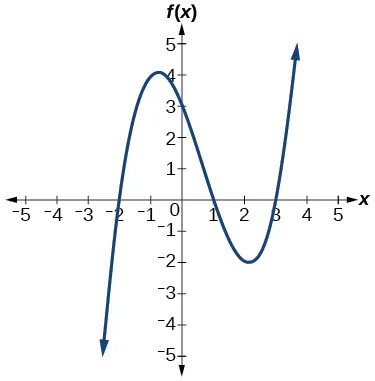49.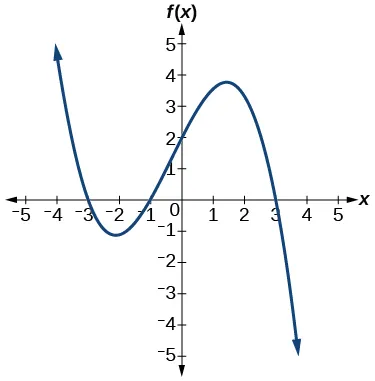50.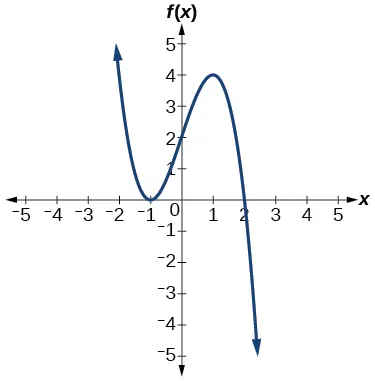51.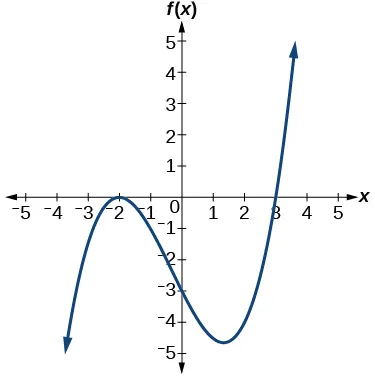52.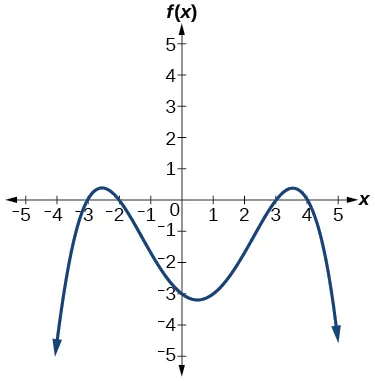For the following exercises, use the graph to identify zeros and multiplicity.

53.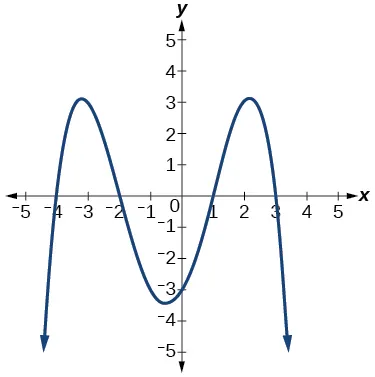54.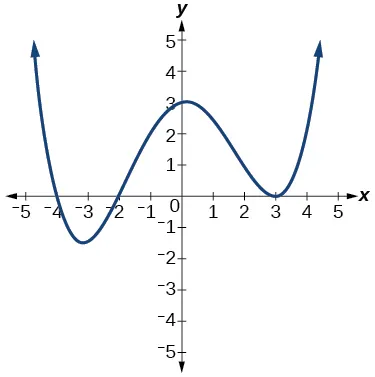55.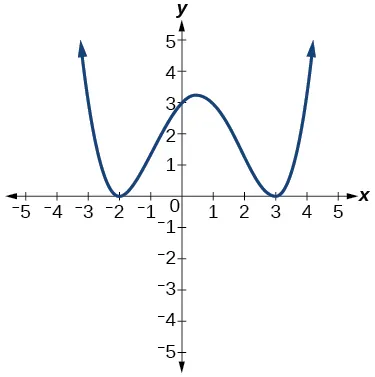56.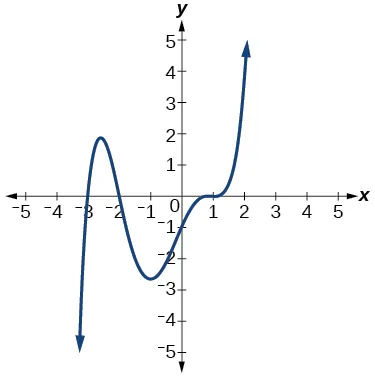For the following exercises, use the given information about the polynomial graph to write the equation.

57.

Degree 3. Zeros at $x=–2, x=–2,$ $x=1, x=1,$ and $x=3. x=3.$ y-intercept at $(0,–4). (0,–4).$

58.

Degree 3. Zeros at $x=–5, x=–5,$ $x=–2, x=–2,$ and $x=1. x=1.$ y-intercept at $(0,6) (0,6)$

59.

Degree 5. Roots of multiplicity 2 at $x=3 x=3$ and $x=1 x=1$ , and a root of multiplicity 1 at $x=–3. x=–3.$ y-intercept at $(0,9) (0,9)$

60.

Degree 4. Root of multiplicity 2 at $x=4, x=4,$ and a roots of multiplicity 1 at $x=1 x=1$ and $x=–2. x=–2.$ y-intercept at $(0,–3). (0,–3).$

61.

Degree 5. Double zero at $x=1, x=1,$ and triple zero at $x=3. x=3.$ Passes through the point $(2,15). (2,15).$

62.

Degree 3. Zeros at $x=4, x=4,$ $x=3, x=3,$ and $x=2. x=2.$ y-intercept at $( 0,−24 ). ( 0,−24 ).$

63.

Degree 3. Zeros at $x=−3, x=−3,$ $x=−2 x=−2$ and $x=1. x=1.$ y-intercept at $(0,12). (0,12).$

64.

Degree 5. Roots of multiplicity 2 at $x=−3 x=−3$ and $x=2 x=2$ and a root of multiplicity 1 at $x=−2. x=−2.$

y-intercept at

65.

Degree 4. Roots of multiplicity 2 at $x= 1 2 x= 1 2$ and roots of multiplicity 1 at $x=6 x=6$ and $x=−2. x=−2.$

y-intercept at $( 0,18 ). ( 0,18 ).$

66.

Double zero at $x=−3 x=−3$ and triple zero at $x=0. x=0.$ Passes through the point $(1,32). (1,32).$

#### Technology

For the following exercises, use a calculator to approximate local minima and maxima or the global minimum and maximum.

67.

$f(x)= x 3 −x−1 f(x)= x 3 −x−1$

68.

$f(x)=2 x 3 −3x−1 f(x)=2 x 3 −3x−1$

69.

$f(x)= x 4 +x f(x)= x 4 +x$

70.

$f(x)=− x 4 +3x−2 f(x)=− x 4 +3x−2$

71.

$f(x)= x 4 − x 3 +1 f(x)= x 4 − x 3 +1$

#### Extensions

For the following exercises, use the graphs to write a polynomial function of least degree.

72.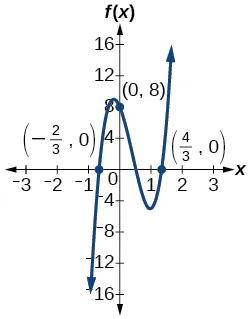73.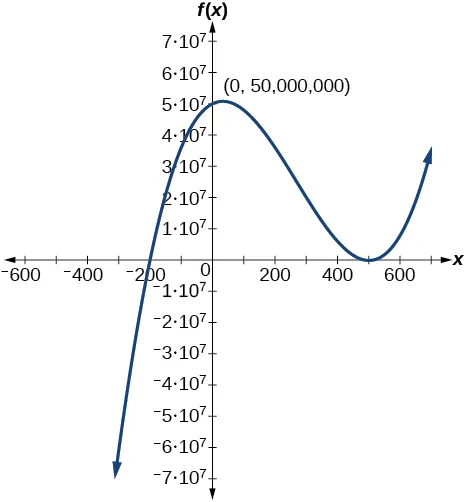74.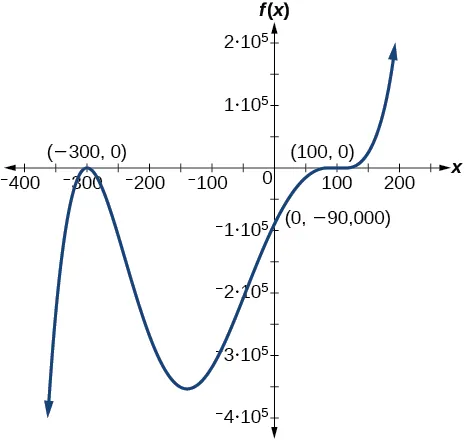#### Real-World Applications

For the following exercises, write the polynomial function that models the given situation.

75.

A rectangle has a length of 10 units and a width of 8 units. Squares of $x x$ by $x x$ units are cut out of each corner, and then the sides are folded up to create an open box. Express the volume of the box as a polynomial function in terms of $x. x.$

76.

Consider the same rectangle of the preceding problem. Squares of $2x 2x$ by $2x 2x$ units are cut out of each corner. Express the volume of the box as a polynomial in terms of $x. x.$

77.

A square has sides of 12 units. Squares $x+1 x+1$ by $x+1 x+1$ units are cut out of each corner, and then the sides are folded up to create an open box. Express the volume of the box as a function in terms of $x. x.$

78.

A cylinder has a radius of $x+2 x+2$ units and a height of 3 units greater. Express the volume of the cylinder as a polynomial function.

79.

A right circular cone has a radius of $3x+6 3x+6$ and a height 3 units less. Express the volume of the cone as a polynomial function. The volume of a cone is $V= 1 3 π r 2 h V= 1 3 π r 2 h$ for radius $r r$ and height $h. h.$

Order a print copy

As an Amazon Associate we earn from qualifying purchases.